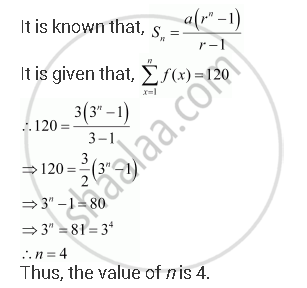Share

# If F is a Function Satisfying F (X +Y) = F(X) F(Y) for All X, Y ∈ N Such That F(1) = 3 and Sum_(X = 1)^N F(X) = 120, Find the Value of N. - Mathematics

#### Question

If f is a function satisfying f (x +y) = f(x) f(y) for all x, y ∈ N such that f(1) = 3 and sum_(x = 1)^n f(x) = 120, find the value of n.

#### Solution

It is given that,

(x + y) = (x) × (y) for all xy ∈ N … (1)

(1) = 3

Taking x = y = 1 in (1), we obtain

f (1 + 1) = (2) = (1) (1) = 3 × 3 = 9

Similarly,

(1 + 1 + 1) = (3) = (1 + 2) = (1) (2) = 3 × 9 = 27

(4) = (1 + 3) = f (1) (3) = 3 × 27 = 81

∴ (1), (2), (3), …, that is 3, 9, 27, …, forms a G.P. with both the first term and common ratio equal to 3.Is there an error in this question or solution?
Solution If F is a Function Satisfying F (X +Y) = F(X) F(Y) for All X, Y ∈ N Such That F(1) = 3 and Sum_(X = 1)^N F(X) = 120, Find the Value of N. Concept: Geometric Progression (G. P.).